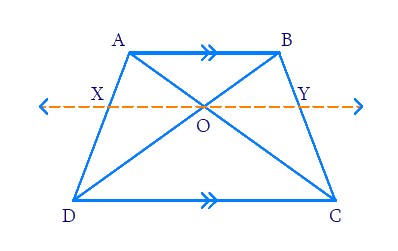# Ex.6.2 Q9 Triangles Solution - NCERT Maths Class 10

Go back to  'Ex.6.2'

## Question

$$ABCD$$ is a trapezium in which $$AB || DC$$ and its diagonals intersect each other at the point $$O.$$ Show that \begin{align}\frac{AO}{BO}=\frac{CO}{DO} \end{align}## Text Solution

Reasoning:

As we know if a line divides any two sides of a triangle in the same ratio, then the line is parallel to the third side.

Steps:

In trapezium $$ABCD$$

$$AB||CD$$ ,$$AC$$ and  $$BD$$ intersect at $$‘O’$$

Construct $$XY$$ parallel to $$AB$$ and $$CD$$ $$(XY\parallel AB,(XY \parallel CD)$$through $$‘O’$$

In \begin{align}\Delta ABC\end{align}

$$OY||AB$$ $$(\because construction)$$

According to theorem $$6.1$$ (BPT)

\begin{align}\frac{BY}{CY}=\frac{OA}{OC}\,\,\,.............\rm{(I)}\end{align}

In $$\Delta BCD$$

$$\rm OY||CD\; (\because\; \rm{construction})$$

According to theorem $$6.1$$ ($$BPT$$)

\begin{align}\frac{BY}{CY}=\frac{OB}{OD}.................\left( \text{II} \right) \\ \end{align}

From $$\rm (I)$$ and $$\rm (II)$$

\begin{align} \,\,\,\,\,\,\frac{OA}{OC}&=\frac{OB}{OD}\,\,\,\\ \Rightarrow\quad \frac{OA}{OB}&=\frac{OC}{OD} \\ \end{align}

Learn from the best math teachers and top your exams

• Live one on one classroom and doubt clearing
• Practice worksheets in and after class for conceptual clarity
• Personalized curriculum to keep up with school